Year 10 Interactive Maths - Second Edition

## Quartiles

If a data set of scores is arranged in ascending order of magnitude, then:
• The median is the middle value of the data set.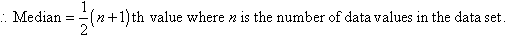• The lower quartile (Q1) is the median of the lower half of the data set.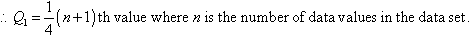• The upper quartile (Q3) is the median of the upper half of the data set.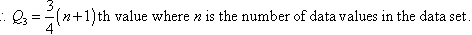• The interquartile range (IQR) is the spread of the middle 50% of the data values. So: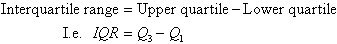The interquartile range is a more useful measure of spread than range as it describes the middle 50% of the data values and thus, is less affected by outliers.

#### Example 6

Find the median, lower quartile, upper quartile and interquartile range of the following data set of scores:

19     21     24     21     24     28     25     24     30

##### Solution:

Arrange the score values in ascending order of magnitude:

19     21     21    24     24     24     25     28    30

There are 9 values in the data set.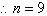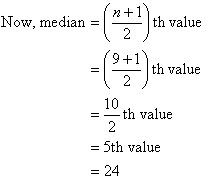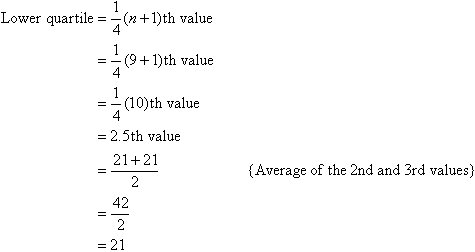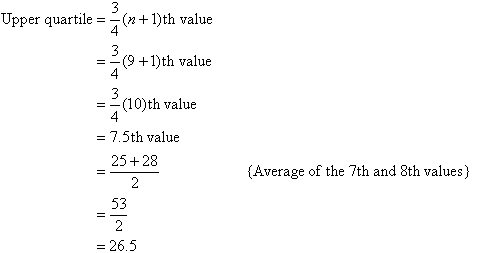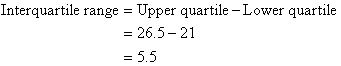This means the middle 50% of the data values range from 21 to 26.5.

###### Note:
• The quartiles divide the set of measurements into four equal parts. Twenty-five per cent of the measurements are less than the lower quartile, fifty per cent of the measurements are less than the median and seventy-five per cent of the measurements are less than the upper quartile. So, fifty per cent of the measurements are between the lower quartile and the upper quartile.
• The lower quartile, median and upper quartile are often denoted by Q1, Q2 and Q3 respectively.
• The median is also denoted by m.